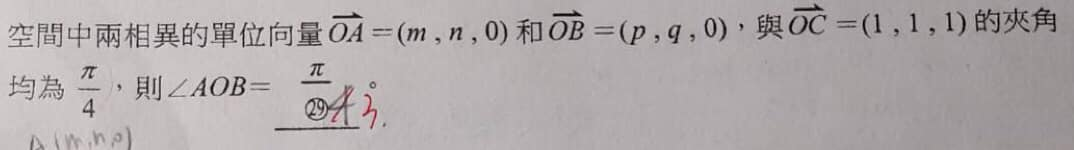# 請問

## 請問TOP

## 請參考附件

#### 附件0116計算.pdf (108.58 KB)

2021-1-16 18:30, 下載次數: 670

TOP

 satsuki931000 satsuki發短消息 加為好友 當前離線 3# 大 中 小 發表於 2021-1-16 23:34  只看該作者 易知 $$m+n=\frac{\sqrt{6}}{2}$$  且  $$m^2+n^2=1$$ 計算可得$$m=\frac{\sqrt{6}+\sqrt{2}}{4},n=\frac{\sqrt{6}-\sqrt{2}}{4}$$ 因為$$\vec{OA} \neq \vec{OB}$$ 所以易知 $$p=\frac{\sqrt{6}-\sqrt{2}}{4},q=\frac{\sqrt{6}+\sqrt{2}}{4}$$ 接下來就簡單了 所求為$$\frac{1}{4}+\frac{1}{4}=1\times 1\times cos\theta$$ $$cos \theta =\frac{1}{2}$$ $$\theta= \frac{\pi}{3}$$ UID2381 帖子293 閱讀權限10 在線時間548 小時 註冊時間2017-3-21 最後登錄2021-12-5  查看詳細資料 TOP

## 回復 3# satsuki931000 的帖子

satsuki931000老師好

pi/3,要怎麼剔除pi/4的答案   謝謝

TOP

 satsuki931000 satsuki發短消息 加為好友 當前離線 5# 大 中 小 發表於 2021-1-17 00:38  只看該作者 您的算式裡面假設$$OA,OB$$夾角45度 但題目應該沒有說他們兩個的夾角 我的解讀是前兩個向量都和$$OC$$夾45度 但 $$OA,OB$$ 夾角是未知的 UID2381 帖子293 閱讀權限10 在線時間548 小時 註冊時間2017-3-21 最後登錄2021-12-5  查看詳細資料 TOP
 weiye 瑋岳發短消息 加為好友 當前離線 6# 大 中 小 發表於 2021-1-17 07:47  只看該作者 設 O 為原點，則 A, B 皆為xy平面上的點， 易知 OC與正向z軸夾角的餘弦值為 1/√3， OC與正向z軸夾角的正弦值為 √2/√3 得 OC 在xy平面的投影長 = (√2/√3)*OC長 = √2 因為 OC 與 OA或OB 夾角皆為 pi/4，所以 OC 在 OA或OB 上的投影長皆為 √3/√2 設 OA 與 OB 夾角為 2 theta， 則 （中間透過三垂線定理） cos theta = (√3/√2)/√2 = √3/2 得 theta = 30度 OA 與 OB 夾角為 60度  =  pi/3。 UID1 帖子2287 閱讀權限200 在線時間7818 小時 註冊時間2006-3-5 最後登錄2021-12-7  查看詳細資料 TOP

TOP

﻿